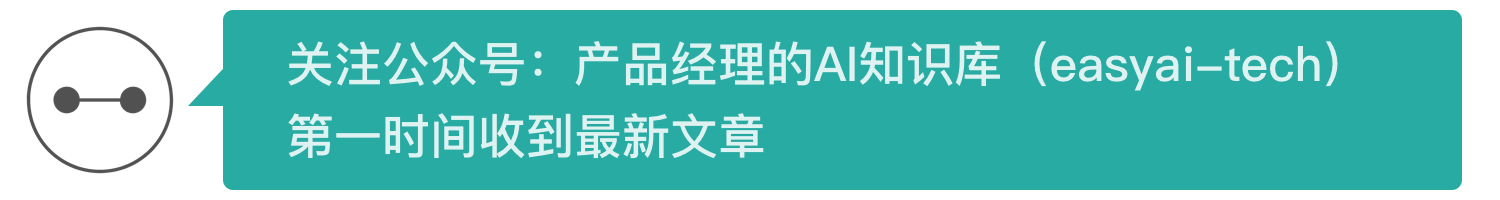In the previous section (How to understand RNN? (Theory)The course introduces the basic structure of the cyclic neural network. At the same time, such a loop structure will bring some difficulties to the optimization. This paper mainly introduces two simple ways to alleviate the problem.RNNOptimization problem:

1. Orthogonal initialization
2. Activation function selection

## Two key points of BPTT

We can write the forward propagation formula in the above figure, using f as the activation function of the hidden unit, g as the activation function of the output unit, in order to simplify the problem, do not use the offset, nor use the threshold in the unit, a circle represents only one Neurons, taking St as an example:

among them:

In the case of backpropagation, we can't use the original layer-by-layer backpropagation method to update the parameters, because the data has different entry order when used, and each time step shares the parameters. We need to consider the update of the individual time steps. Information on the entire sequence. We call this the way of BP through time, but the real essential mechanism is only two, parameter sharing and loop structure.

To understand this is not difficult, we only need to consider matrix multiplication, when the parameters are not shared, from the formal point of view, matrix parameter updates do not interfere with each other, we can easily update each parameter, but if Parameter sharing, then the elements of the matrix need to be bound together to update, the update of the gradient becomes the sum of the gradients of the regions shared by the parameters. Because the area shared by the parameters is shared along time, summation also needs to follow time. (in the same parameter sharingCNNIn the back propagation, the sum is in accordance with the space)

For the update of the parameter V, we must consider the parameter sharing at different time steps. We can write the forward propagation as a matrix form:

There are:On this basis, we perform the same forward propagation operation on the parameter U:

We will find that the variable St of the current time step will contain the variable St-1 of the previous step. At this time, we choose to evaluate the gradient of U or W. We cannot ignore the variable of the previous step because the variable of the previous step also contains the parameter. U and W, then when we update U, we need to recursively expand the previous time step:

because:

In the same way, we update the parameters of W, which is the same structure:

We can clearly see that the weight sharing mechanism makes us need to sum each time step gradient, the loop structure makes us need to recursively process the gradient, according to our expansion, each

Will produce one or more W, such as:

As our chain grows longer, the entire chain appears to be multiplied by W, which is what the loop structure brings.

In ordinary neural networks, gradient disappearance often comes from activation functions and layer-to-layer coordination updates, but in RNN, one of the sources of gradient disappearance and explosion is the sharing of shared parameters W. In RNN, if there is no gradient flow in the loop layer, then the information of the sequence is not conveyed. Our so-called memory unit will lose its memory ability, and the advantage of RNN compared with the traditional n-gram model will cease to exist. .

In theory, we want to keep the W value as close as possible, so that the network can be effectively trained.

## Orthogonal initialization

The idea of ​​orthogonal initialization is very simple, that is, the nature of the orthogonal matrix is ​​utilized, and its transposed matrix is ​​its inverse matrix, which has:

The multiplication of the matrix does not enlarge or reduce the value of W itself.

We can also understand from another angle that if we do eigenvalue decomposition on the matrix, we decompose it into the product of the diagonal matrix and the orthogonal matrix:

The multiplication of the matrix becomes:

The multiplication of the matrix becomes a multiplication of its eigenvalues, so:

• If the absolute value of the eigenvalue is less than 1, then the parameter gradient will become smaller and smaller.

• If the eigenvalues ​​are approximately equal to 1, then the parameter gradient can maintain the normal range.

• If the absolute value of the eigenvalues ​​is greater than 1, then the parameter gradient will become larger and larger.

The eigenvalues ​​of the orthogonal matrix are either 1 or -1, although we cannot guarantee the parameters.matrixW is always in this form, but at least it can do this on initialization.

## Activation function

We have long talked about the importance of activation functions. The sigmoid function has decentralization and extensive saturation. ReLU and its various variants can solve this problem very well, but in the cyclic neural network, we need Considering the eigenvalue of the parameter, ReLU is a linear function at its right end. Although its gradient is constant 1, the function value can grow indefinitely.

The traditional ReLU may bring the problem of gradient explosion in RNN, but we usually adopt the clipping method to solve the gradient explosion, that is, if the gradient is larger than a certain value, it is equal to a certain value. In addition, we can also use the tanh activation function, because its range is [-1, 1], better adapt to the gradient explosion caused by parameter multiplication, but also to some extent can not avoid the gradient disappear.

We need to do a trade-off between the two because we don't want the activation function to make the weighted part too big or too small, nor do we want the value of the function itself to be too big or too small.